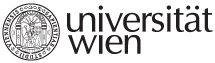Name of course DXE_EMTR Econometrics Lecturer Prof. Dr. Peter Hackl Assistant Ing. Daniel Němec, Ph.D. ECTS 12 credits Extent and Intensity 24 teaching hours, 45 minutes each (6 lectures á 4 hours) Schedule 5.10., 26.10., 2.11., 9.11., 23.11., 30.11; 10:00–16:00 Course objectives The course introduces students to common used econometric tools and techniques. Students shall gain sufficient knowledge and experience for his/her independent and qualified work with empirical data. The student should be able to formulate correctly, to identify economic models and to interpret the results accordingly. Prerequisites Participants should be familiar with the following topics: • Linear algebra – linear equations, matrices, vectors (basic operations and properties). • Descriptive statistics – measures of central tendency, measures of dispersion, measures of association, histogram, frequency tables, scatterplot, quantiles • Theory of probability – probability and its properties, random variables and distribution functions in one and several dimensions, moments, convergence of random variables, limit theorems, law of large numbers. • Mathematical statistics – point estimation, confidence intervals for parameters of normal distribution, hypothesis testing, p-value, significance level. • These topics correspond to the appendices of Verbeek’s book, in particular, to the sections: A1, A2, A3, A4, A6, A8, B1, B2, B3 (excluding Jensen's inequality), B4, B5, B6 and B7 (excluding some properties of the chi-squared distribution and the F-distribution) Syllabus 1. Introduction to linear regression model (Verbeek, Ch. 2) •         normal linear regression model •         least squares method •         properties of OLS estimators 2. Introduction to linear regression model (Verbeek, Ch. 2) •         goodness of fit •         hypotheses testing •         multicollinearity 3. Interpreting and comparing regression models (Verbeek, Ch.3) •         interpretation of the fitted model •         selection of regressors •         testing the functional form 4. Heteroskedascity and autocorrelation (Verbeek, Ch. 4) •         causes, consequences, testing, alternatives for inference 5. Endogeneity, instrumental variables and GMM (Verbeek, Ch. 5) •         the instrumental variables estimator •         the generalized instrumental variables estimator •         the Generalized Method of Moments (principles and examples of use) 6. The practice of econometric modeling Literature VERBEEK, Marno. A guide to modern econometrics. 4th ed. Chichester: John Wiley & Sons, 2012. xv, 497. ISBN 9781119951674. KENNEDY, Peter. A guide to econometrics. 6th ed. Malden: Blackwell, 2008. xii, 585. ISBN 9781405182584. Teaching methods Class discussion, homework including computer exercises using Gretl, and presentation of homework by participants; course language is English. Assessment methods For grading, written homework, presentation of homework in class and a final written exam will be of relevance. The weights are as follows: homework with 40%, final exam (consisting of theoretical and practical part) with 60%. The presentation of homework in class means that students must be prepared to be called at random. Minimal requirements to pass final exam are as follows: 60%.
University of Vienna | Universitätsring 1 | 1010 Vienna | T +43-1-4277-0